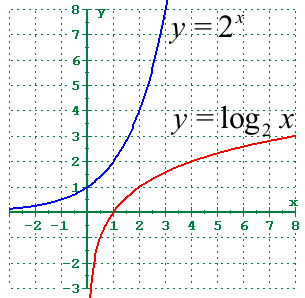# 4.2 - Logarithmic Functions and Their Graphs

## Inverse of Exponential FunctionsWe stated in the section on exponential functions, that exponential functions were one-to-one. One-to-one functions had the special property that they have inverses that are also functions. And, as many of you said in class, and I'm so glad you remember, one-to-one functions can be applied to both sides of an equation. They also pass a horizontal line test.

This section is about the inverse of the exponential function. The inverse of an exponential function is a logarithmic function. Remember that the inverse of a function is obtained by switching the x and y coordinates. This reflects the graph about the line y=x. As you can tell from the graph to the right, the logarithmic curve is a reflection of the exponential curve.

The table below demonstrates how the x and y values of the points on the expontential curve can be switched to find the coordinates of the points on the logarithmic curve.

Point on
exponential curve
Corresponding point
on logarithmic curve
(-3, 1/8) (1/8, -3)
(-2, 1/4) (1/4, -2)
(-1, 1/2) (1/2, -1)
(0, 1) (1, 0)
(1, 2) (2, 1)
(2, 4) (4, 2)
(3, 8) (8, 3)

### Comparison of Exponential and Logarithmic Functions

Let's look at some of the properties of the two functions.

The standard form for a logarithmic function is: y = loga x

Note, if the "a" in the expression above is not a subscript (lower than the "log"), then you need to update your web browser.

Exponential Logarithmic
Function y=ax, a>0, a≠1 y=loga x, a>0, a≠1
Domain all reals x > 0
Range y > 0 all reals
intercept y = 1 x = 1
increasing when a > 1 when a > 1
decreasing when 0 < a < 1 when 0 < a < 1
asymptote x-axis (y=0) y-axis (x=0)
continuous yes yes
smooth yes yes

## Working Definition of Logarithm

In the exponential function, the x was the exponent. The purpose of the inverse of a function is to tell you what x value was used when you already know the y value. So, the purpose of the logarithm is to tell you the exponent.

Thus, our simple definition of a logarithm is that it is an exponent.

Another way of looking at the expression "loga x" is "to what power (exponent) must a be raised to get x?"

## Equivalent Forms

The logarithmic form of the equation y=loga x is equivalent to the exponential form x=ay.

To rewrite one form in the other, keep the base the same, and switch sides with the other two values.

## Properties of Logarithms

loga 1 = 0 because a0 = 1
No matter what the base is, as long as it is legal, the log of 1 is always 0. That's because logarithmic curves always pass through (1,0)
loga a = 1 because a1 = a
Any value raised to the first power is that same value.
loga ax = x
The log base a of x and a to the x power are inverse functions. Whenever inverse functions are applied to each other, they inverse out, and you're left with the argument, in this case, x.
loga x = loga y implies that x = y
If two logs with the same base are equal, then the arguments must be equal.
loga x = logb x implies that a = b
If two logarithms with the same argument are equal, then the bases must be equal.

## Common Logs and Natural Logs

There are two logarithm buttons on your calculator. One is marked "log" and the other is marked "ln". Neither one of these has the base written in. The base can be determined, however, by looking at the inverse function, which is written above the key and accessed by the 2nd key.

### Common Logarithm (base 10)

When you see "log" written, with no base, assume the base is 10.
That is: log x = log10 x.

Some of the applications that use common logarithms are in pH (to measure acidity), decibels (sound intensity), the Richter scale (earthquakes).

An interesting (possibly) side note about pH. "Chapter 50: Sewers" of the Village of Forsyth Code requires forbids the discharge of waste with a pH of less than 5.5 or higher than 10.5 (section 50.07).

Common logs also serve another purpose. Every increase of 1 in a common logarithm is the result of 10 times the argument. That is, an earthquake of 6.3 has 10 times the magnitude of a 5.3 earthquake. The decibel level of loud rock music or a chainsaw (115 decibels = 11.5 bels) is 10 times louder than chickens inside a building (105 decibels = 10.5 bels)

### Natural Logarithms (base e)

Remember that number e, that we had from the previous section? You know, the one that was approximately 2.718281828 (but doesn't repeat or terminate). That is the base for the natural logarithm.

When you see "ln" written, the base is e.
That is: ln x = loge x

Exponential growth and decay models are one application that use natural logarithms. This includes continuous compounding, radioactive decay (half-life), population growth. Typically applications where a process is continually happening. Now, these applications were first mentioned in the exponential section, but you will be able to solve for the other variables involved (after section 4) using logarithms.

In higher level mathematics, the natural logarithm is the logarithm of choice. There are several special properties of the natural logarithm function, and it's inverse function, that make life much easier in calculus.

Since "ln x" and "ex" are inverse functions of each other, any time an "ln" and "e" appear right next to each other, with absolutely nothing in between them (that is, when they are composed with each other), then they inverse out, and you're left with the argument.